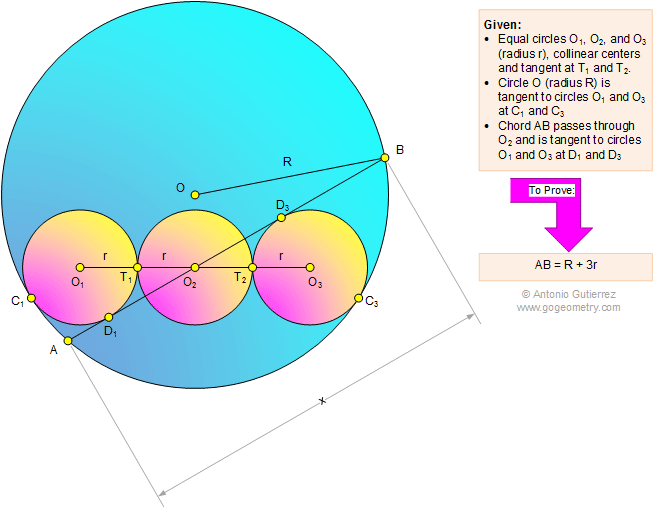Problem 1096: Tangent Circles, Common Tangent, Chord, Radius, Center. Level: High School, SAT Prep, College Geometry

< PREVIOUS PROBLEM  |  NEXT PROBLEM >

 The figure below shows the equal circles O1, O2, and O3 (radii r) with collinear centers and tangent at T1 and T2. Circle O (radius R) is tangent to circles O1 and O3 at C1 and C3, respectively. Chord AB passes through O2 and is tangent to circles O1 and O3 at D1 and D3, respectively. Prove that AB = R + 3r.Home | Search | Geometry | Problems | All Problems | Open Problems | Visual Index | 1091-1100 | Circle | Tangent Line | Tangent Circles | Chord | Email | Post a comment or solution | By Antonio GutierrezLast updated: Mar 11, 2015